# 基于BP神经网络的压电智能旋翼主动减振仿真分析Simulation Analysis of Active Vibration Reduction of Piezoelectric Intelligent Rotor Based on BP Neural Network

DOI: 10.12677/MOS.2021.101006, PDF, HTML, XML, 下载: 73  浏览: 376

Abstract: In order to improve the ability of search and rescue aircraft to maintain stable operation even under sudden external interference, an intelligent rotor structure is designed based on the piezo-electric composite material that uses its own adjustment mechanism to quickly respond and adapt to external impacts to achieve active vibration control method; piezoelectric intelligent rotor active vibration reduction model has been established based on PID control method of BP neural network algorithm; Achieve real-time control of the aircraft during flight by adjusting dynamically yaw angle, roll angle and pitch angle using BP Network, using Simulink module. Finally, a control simulation test is carried out considering the actual flight control state. The results show that the active vibration reduction control method has a feedback control function with disturbance compensation, which can effectively suppress the vibration of the rotor during flight, and the vibration reduction effect can be increased by 15%~30%.

1. 引言

2. 压电智能旋翼结构的耦合模型

2.1. 数值模型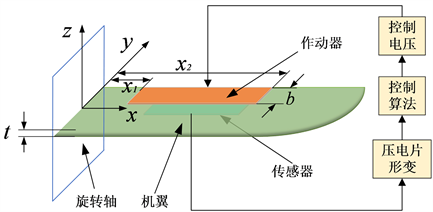Figure 1. Piezoelectric intelligent rotor structure

${E}_{a}{I}_{a}\frac{{\partial }^{4}\omega }{\partial {x}^{4}}+{\rho }_{a}{A}_{a}\frac{{\partial }^{2}\omega }{\partial {t}^{2}}-b{\sum }_{i=1}^{m}\frac{{\partial }^{2}{M}_{i}^{e}}{\partial {x}^{2}}=0$ (1)

${E}_{a}{I}_{a}\frac{{\partial }^{4}\omega }{\partial {x}^{4}}+{\rho }_{a}{A}_{a}\frac{{\partial }^{2}\omega }{\partial {t}^{2}}={\sum }_{i=1}^{m}{k}_{a}{U}_{i}^{e}\left[\stackrel{¨}{H}\left(x-{x}_{i2}\right)-\stackrel{¨}{H}\left(x-{x}_{i1}\right)\right]$ (2)

$\omega \left(x,t\right)={\sum }_{j=1}^{n}{\phi }_{j}\left(x\right){\eta }_{j}\left(t\right)$ (3)

${\stackrel{¨}{\eta }}_{j}\left(t\right)+2{\xi }_{j}{\omega }_{j}{\stackrel{˙}{\eta }}_{j}+{\omega }_{j}^{2}{\eta }_{j}\left(t\right)={\sum }_{i=1}^{m}{k}_{a}\left[{\stackrel{˙}{\phi }}_{j}\left({x}_{i2}\right)-{\stackrel{˙}{\phi }}_{j}\left({x}_{i1}\right)\right]{U}_{i}^{\theta }\left(t\right)$ (4)

$\left\{\begin{array}{l}\stackrel{˙}{X}=AX+B{U}_{a}\\ y={U}_{s}=CX\end{array}$ (5)

2.2. 动力学模型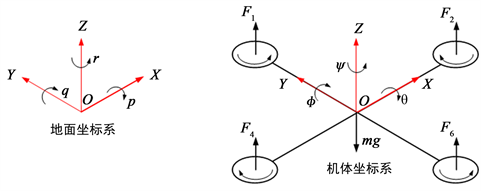Figure 2. Ground reference coordinate system and Airframe coordinate system

$\begin{array}{l}R=\left[\begin{array}{ccc}\mathrm{cos}\psi & -\mathrm{sin}\psi & 0\\ \mathrm{sin}\psi & \mathrm{cos}\psi & 0\\ 0& 0& 1\end{array}\right]×\left[\begin{array}{ccc}\mathrm{cos}\theta & 0& \mathrm{sin}\theta \\ 0& 1& 0\\ -\mathrm{sin}\theta & 0& \mathrm{cos}\theta \end{array}\right]×\left[\begin{array}{ccc}1& 0& 0\\ 0& \mathrm{cos}\varphi & -\mathrm{sin}\varphi \\ 0& \mathrm{sin}\varphi & \mathrm{cos}\theta \end{array}\right]\\ \text{ }\text{ }\text{ }\text{ }\text{ }\text{ }\text{ }=\left[\begin{array}{ccc}\mathrm{cos}\theta \mathrm{cos}\psi & cos\psi \mathrm{sin}\theta \mathrm{sin}\varphi -\mathrm{sin}\psi \mathrm{cos}\varphi & \mathrm{sin}\varphi \mathrm{sin}\psi +\mathrm{cos}\psi \mathrm{sin}\theta \mathrm{cos}\varphi \\ \mathrm{sin}\psi & cos\theta \mathrm{cos}\psi +\mathrm{sin}\theta \mathrm{sin}\varphi \mathrm{sin}\psi & \mathrm{sin}\psi \mathrm{sin}\varphi \mathrm{sin}\psi -\mathrm{cos}\psi \mathrm{sin}\theta \\ -\mathrm{sin}\theta & \mathrm{sin}\varphi cos\theta & cos\theta cos\varphi \end{array}\right]\end{array}$ (6)

$\begin{array}{l}{F}_{B}={\left[{F}_{x},{F}_{y},{F}_{z}\right]}^{\text{T}}={\left[0,0,{U}_{1}\right]}^{\text{T}}\\ {U}_{1}={F}_{1}+{F}_{2}+{F}_{3}+{F}_{4}\end{array}$ (7)

${F}_{G}=R{F}_{B}=R{\left[{F}_{x},{F}_{y},{F}_{z}\right]}^{\text{T}}$ (8)

$\left\{\begin{array}{l}\stackrel{¨}{x}=\frac{{U}_{1}\left(\mathrm{sin}\varphi \mathrm{sin}\psi +\mathrm{cos}\psi \mathrm{sin}\theta \mathrm{cos}\varphi \right)}{m}\\ \stackrel{¨}{y}=\frac{{U}_{1}\left(\mathrm{sin}\psi \mathrm{sin}\theta \mathrm{cos}\varphi -\mathrm{cos}\psi \mathrm{sin}\varphi \right)}{m}\\ \stackrel{¨}{z}=\frac{{U}_{1}\mathrm{cos}\varphi \mathrm{cos}\theta }{m}-g\end{array}$ (9)

$\left\{\begin{array}{l}\stackrel{¨}{\varphi }=\frac{{U}_{2}}{{I}_{x}}=\frac{kl\left({w}_{4}^{2}-{w}_{2}^{2}\right)}{{I}_{x}}\\ \stackrel{¨}{\theta }=\frac{{U}_{3}}{{I}_{y}}=\frac{kl\left({w}_{3}^{2}-{w}_{1}^{2}\right)}{{I}_{x}}\\ \stackrel{¨}{\psi }=\frac{{U}_{4}}{{I}_{z}}=\frac{kl\left(-{w}_{1}^{2}+{w}_{2}^{2}-{w}_{3}^{2}+{w}_{4}^{2}\right)}{{I}_{z}}\end{array}$ (10)

3. 基于神经网络的PID主动减振控制系统

3.1. 控制系统原理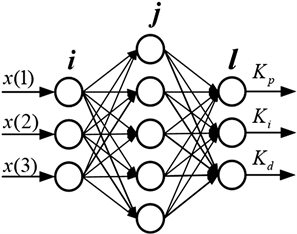Figure 3. BP Neural Network Structure diagram

$\begin{array}{l}{x}_{1}\left(t\right)=e\left(t\right)=r\left(t\right)-y\left(t\right)\\ {x}_{2}\left(t\right)=\Delta e\left(t\right)=e\left(t\right)-e\left(t-1\right)\\ {x}_{3}\left(t\right)={\Delta }^{2}e\left(t\right)=e\left(t\right)-2e\left(t-1\right)+e\left(t-2\right)\end{array}$ (11)

$\Delta u\left(t\right)={k}_{1}{x}_{1}\left(t\right)+{k}_{2}{x}_{2}\left(t\right)+{k}_{3}{x}_{3}\left(t\right)$ (12)

$J=\frac{1}{2}{\left(r\left(t+1\right)-y\left(k+1\right)\right)}^{2}$ (13)

$\frac{\partial J}{\partial \omega }=-\lambda \frac{\partial J}{\partial y\left(t+1\right)}\cdot \frac{\partial y\left(t+1\right)}{\partial u\left(t\right)}\cdot \frac{\partial u\left(t\right)}{\partial \omega }$ (14)

$\left\{\begin{array}{l}\Delta {\omega }_{jl}^{3}\left(t\right)=\alpha \Delta {\omega }_{jl}^{3}\left(t-1\right)+\eta {\delta }_{l}^{3}{O}_{j}^{2}\left(t\right)\hfill \\ \begin{array}{l}{\delta }_{l}^{3}=e\left(t\right)sgn\left(\frac{\partial y\left(t+1\right)}{\partial u\left(t\right)}\right)\cdot \frac{\partial u\left(t\right)}{\partial {O}_{l}^{3}\left(t\right)}\cdot {g}^{\prime }\left(ne{t}_{l}^{3}\left(t\right)\right)\\ {\delta }_{i}^{2}\left(t\right)={f}^{\prime }\left(ne{t}_{j}^{2}\left(t\right)\right)\underset{l=1}{\overset{3}{\sum }}{\delta }_{l}^{3}{\omega }_{jl}^{3}\left(t\right)\\ f\left(x\right)=\frac{\mathrm{exp}\left(x\right)-\mathrm{exp}\left(-x\right)}{\mathrm{exp}\left(x\right)+\mathrm{exp}\left(-x\right)}\\ g\left(x\right)=\frac{\mathrm{exp}\left(x\right)}{\mathrm{exp}\left(x\right)+\mathrm{exp}\left(-x\right)}\end{array}\hfill \end{array}$ (15)

NNI辨识器输入层为：

$\left\{\begin{array}{l}u\left(t\right),u\left(t-1\right),\cdots ,u\left(t-{n}_{u}+1\right)\\ y\left(t\right),y\left(t-1\right),\cdots ,y\left(t-{n}_{y}+1\right)\end{array}$ (16)

$J=\frac{1}{2}{\left(y\left(t+1\right)-\stackrel{^}{y}\left(k+1\right)\right)}^{2}$ (17)

3.2. 主动减振控制系统

PID控制采用增量式数字PID控制，表达式如下所示：

$\begin{array}{l}u\left(t\right)=u\left(t-1\right)+\Delta u\left(t\right)\\ u\left(t\right)=u\left(t-1\right)+{K}_{p}\left[e\left(t\right)-e\left(t-1\right)\right]+{K}_{i}e\left(t\right)+{K}_{d}\left[e\left(t\right)-2e\left(k-1\right)+e\left(t-2\right)\right]\end{array}$ (18)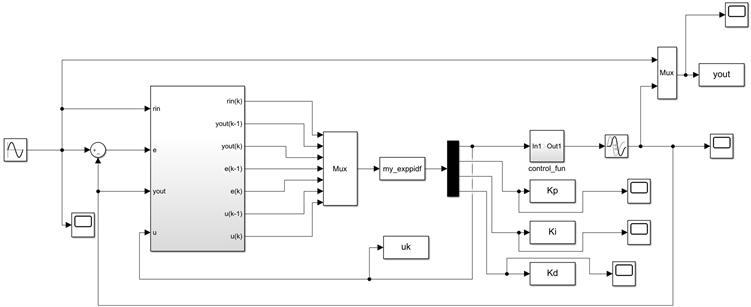Figure 4. Control scheme of flying height based on BP neural network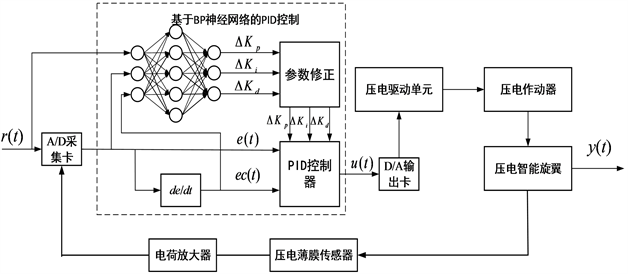Figure 5. Schematic diagram of PID control structure based on BP neural network

4. 主动减振仿真结果及讨论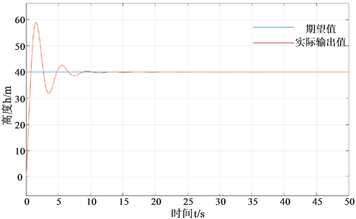(a) 普通PID控制(b) 经BP神经网络算法优化后的控制

Figure 6. Two algorithm control height response graphs without disturbance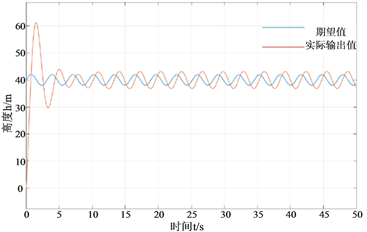(a) 普通PID控制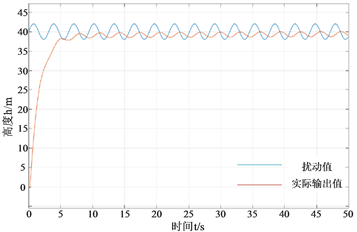(b) 经BP神经网络算法优化后的控制

Figure 7. Two algorithm control height response graphs with disturbance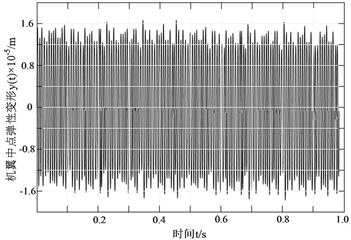(a) 频率为10 HZ且无控制时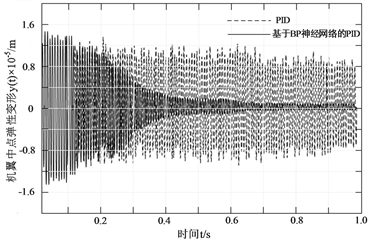(b) 频率为10 HZ时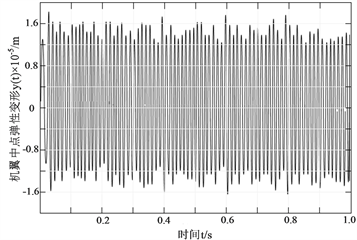(c) 频率为20 HZ且无控制时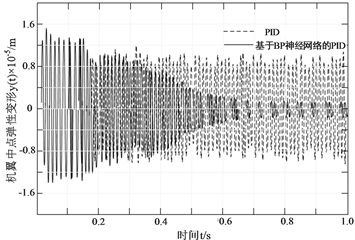(d) 频率为20 HZ时

Figure 8. The y(t) curve diagram of two algorithm control under different frequency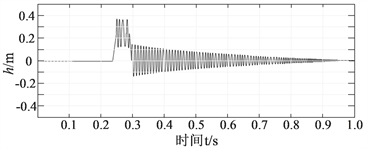(a) 沉浮运动的时间历程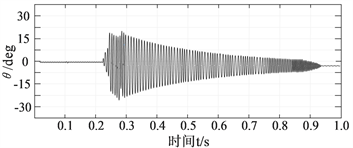(b) 俯仰角运动的时间历程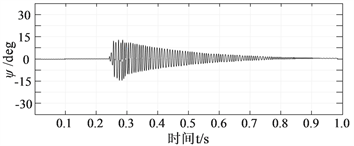(c) 偏转角运动的时间历程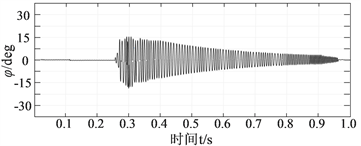(d) 横滚角运动的时间历程

Figure 9. Consider the oscillating response graph under actual flight conditions

5. 结论

NOTES

*通讯作者。

  戴良景, 周国庆. 主动扭转智能旋翼频域研究及仿真[J]. 沈阳航空工业学院学报, 2006, 23(4): 9-11.  田翔, 沈亚娟, 马小艳. 旋翼主动控制技术研究[J]. 中国科技信息, 2019(Z1): 37-44.  Bailey, T. and Hubbard, J.E.J. (1985) Distributed Piezoelectric-Polymer Active Vibration Control of a Cantilever Beam. Journal of Guidance Control & Dynamics, 8, 23. https://doi.org/10.2514/3.20029  Hu, Y.R. and Ng, A. (2005) Active Robust Vibration Control of Flexible Structures. Journal of Sound & Vibration, 288, 43-56. https://doi.org/10.1016/j.jsv.2004.12.015  丛聪, 崔锐, 殷鑫. 风力机叶片面内振动的主动控制研究[J]. 可再生能源, 2017, 35(8): 1236-1243.  Brillante, C., Morandini, M. and Mantegazza, P. (2016) Periodic Controllers for Vibration Reduction Using Actively Twisted Blades. Aeronautical Journal, 120, 1763-1784. https://doi.org/10.1017/aer.2016.80  苑凯华, 邱志平. 压电复合材料壁板颤振的控制[J]. 北京航空航天大学学报, 2009, 35(12): 1429-1433.  张凤云. 基于压电纤维复合材料的悬臂梁扭转控制研究[D]: [硕士学位论文]. 大连: 大连理工大学2015.  杨正岩, 张佳奇, 高东岳, 等. 航空航天智能材料与智能结构研究进展[J]. 航空制造技术, 2017, 60(17): 36-48.  陆轶, 顾仲权. 直升机结构响应主动控制作动器优化设计研究[J]. 振动与冲击, 2007(3): 26-29+157.